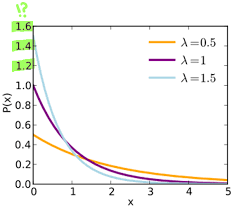## How to Calculate and Solve for P(not A) | ProbabilityThe image above represents P(not A).

To compute for P(not A), two essential parameters are needed and these parameters are Number of Times in Which Event A Can Occur (x) and Total Number of All Possible Outcomes (N).

The formula for calculating P(not A):

P(not A) = (N – x)N

Where;

x = Number of Times in Which Event A can Occur
N = Total Number of All Possible Outcomes

Let’s solve an example;
Find the P(not A) when the number of times in which event A can occur is 7 and the total number of all possible outcomes is 11.

This implies that;

x = Number of Times in Which Event A can Occur = 7
N = Total Number of All Possible Outcomes = 11

P(not A) = (N – x) N
P(not A) = (11 – 7) 11
P(not A) = 4 11
P(not A) = 0.36

Therefore, the P(not A) is 0.36.